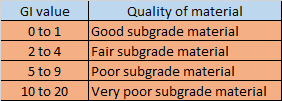## Translate

### VARIATION OF AIR DENSITY WITH ALTITUDE

As fluid properties such as pressure and temperature varies non uniformly with the variation of the altitude, in result the density of air also varies nonuniformly. In lower atmosphere with increase in altitude the temperature and pressure of air decreases but as altitude increases the temperature and pressure varies non uniformly and so the density varies with altitude. The variation  of properties follows following Atmospheric model:-

## (ENGLISH UNIT)

H- altitude(ft) ,  T- temperature(℉)  , P - pressure(lbs/sq.ft) , ρ -density(slugs/cu.ft)

## ρ= P/(1718*(T+459.7))

### 1. TROPOSPHERE REGION (H<36152 ft)

T = 59-0.00356H  i.e. Temperature decreases linearly
P = 2116*((T+459.7)/518.6)^ 5.256 i.e. Pressure decreases exponentially

### 2. LOWER STRATOSPHERE REGION (36152<H<82345)

T = -70 i.e. Temperature remains constant
P = 473.1 * e^(1.73-0.000048H) i.e. Pressure decreases exponentially

### 3.UPPER STRATOSPHERE REGION(H>82345)

T= -205.05+0.00164H i.e. Temperature increases linearly
P= 51.97*((T+459.7)/389.98)^(-11.388) i.e. Pressure decreases exponentially

## (SI UNITS)

H- altitude(m) ,  T- temperature(℃)  , P - pressure(Kg/cu.m) , ρ -density(K-Pa)

ρ= P/(0.2869*(T+273.1))

### 1. TROPOSPHERE REGION (H<11000)

T=15.04 - 0.00649H
P= 101.29*((T+273.1)/288.08)^5.256

### 2. LOWER STRATOSPHERE REGION (11000<H<25000)

T= -56.46
P = 22.65*e^(1.73-0.000157H)

### 3.UPPER STRATOSPHERE REGION(H>25000)

T= -131.21 + 0.00299H
P = 2.488* ( (T+273.1)/216.6) ^(-11.388)

1.2.This post is gives very useful tips for us which is effective our thoughts. Define step by step process that easily understand by us instrumentation valves If you wants more related services then must visit Oshwin Overseas.

3.I found your blog on Google and read a few of your other posts. I just added you to my Google News Reader. You can also visit Carbon steel pipe fittings manufacturers in India for more Rajendra Piping & Fittings related information and knowledge, Keep up the great work Look forward to reading more from you in the future.

### Group index of soil

Group index of soil is a number used to refer the quality of soil used as a subgrade material in highway construction.  Group index of soil ...